Math resources Geometry Volume

Volume of a rectangular prism

# Volume of a rectangular prism

Here you will learn about the volume of a rectangular prism, including what it is and how to find it.

Students will first learn about the volume of a rectangular prism as part of geometry in 5 th grade. Students will expand on this knowledge in 6 th grade to include rectangular prisms with fractional dimensions.

## What is volume of a rectangular prism?

The volume of a rectangular prism is the amount of space there is within it. Since rectangular prisms are 3 dimensional shapes, the space inside them is measured in cubic units.

\text{Volume of a rectangular prism }=\text { length } \times \text { width } \times \text { height }

For example,

This rectangular prism is made from 24 unit cubes – each side is 1 \, cm . That means the space within the rectangular prism, or the volume, is 24 \, \mathrm{cm}^3.

Even though you can’t see all 24 unit cubes, you can prove there are 24 by thinking about the rectangular prism in parts.

The bottom part of the prism is made up by 2 rows of 4 cubes – or 8 total cubes. The bottom part has a volume of 8 \, \mathrm{cm}^3 .

The other layers of the rectangular prism are exactly the same. Since the height is 3 \, cm , there are 3 layers of cubes. Each layer has a volume of 8 \, \mathrm{cm}^3 , so add them to find the total volume.

8 \, cm^3 + 8 \, cm^3 + 8 \, cm^3

V=24 \, cm^3

Thinking about it this further, the volume of a rectangular prism is the area of the base times the height. Consider the same rectangular prism. If you were to hold it up and look at the bottom, it would look like this:

4 \, cm \times 2 \, cm

Area of the base = 8 \, cm^2

And the height of the prism is 3 \, cm , so 8 \, \mathrm{cm}^2 \times 3 \mathrm{~cm}=24 \mathrm{~cm}^3 .

This is why the formula for the volume of a rectangular prism is:

\text{Volume of a rectangular prism } = \text { length } \times \text { width } \times \text { height }

### What is volume of a rectangular prism?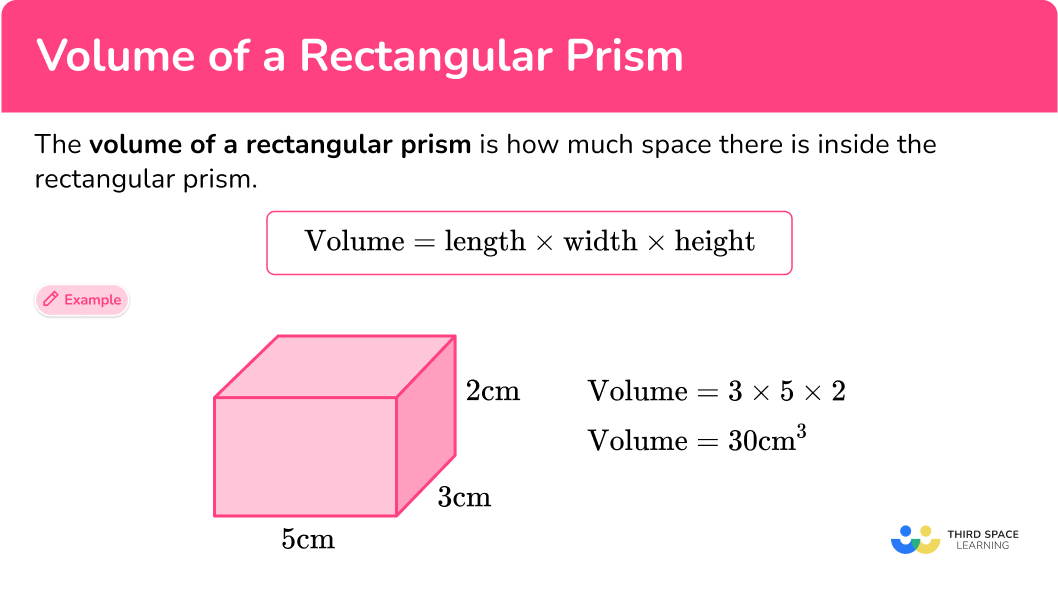## Common Core State Standards

How does this relate to 5 th grade math and 6 th grade math?

• Grade 5 – Geometry (5.G.C.5b)
Apply the formulas V=l \times w \times h and V=b \times h for rectangular prisms to find volumes of right rectangular prisms with whole- number edge lengths in the context of solving real world and mathematical problems.
• Grade 6 – Geometry (6.G.A.2)
Find the volume of a right rectangular prism with fractional edge lengths by packing it with unit cubes of the appropriate unit fraction edge lengths, and show that the volume is the same as would be found by multiplying the edge lengths of the prism.

Apply the formulas V = l \times w \times h and V = b \times h to find volumes of right rectangular prisms with fractional edge lengths in the context of solving real-world and mathematical problems.

## How to calculate the volume of a rectangular prism

In order to find the volume of a rectangular prism with cube units shown:

1. Decide how many cubes make up the first layer.
2. Find the total of all the layers.
3. Write the answer and include the units.

In order to calculate the volume of a rectangular prism with the formula:

1. Write down the formula.
2. Substitute the values into the formula.
3. Solve the equation.
4. Write the answer and include the units.

## Volume of a rectangular prism examples

### Example 1: volume of a rectangular prism

Each cube has a side length of 1 \, inch . Find the volume of the rectangular prism.

1. Decide how many cubes make up the first layer.

If you picked up the prism and looked at the bottom, you would see a 9 by 2 rectangle:

The bottom layer of the rectangular prism is 9 by 2 cubes, so it is made up of 18 inch cubes or 18 \text { inches}{ }^3.

2Find the total of all the layers.

The height is 2 \, inches , so there are 2 layers of cubes.

Since each layer has a volume of 18 \text { inches}^3 , you can add the volume of each layer to find the total volume.

\begin{aligned} \text { Volume } & = 18 \, \text {inches}^3 + 18 \, \text {inches}^3 \\\\ & = 36 \, \text {inches}^3\end{aligned}

You can also multiply the area of the base ( \text {length } \times \text { width} ) times the \text { height} .

\begin{aligned} \text { Volume } & =18 \text { inches}^2 \times 2 \text { inches } \\\\ & =36 \text { inches}^3 \end{aligned}

3Write the answer and include the units.

The dimensions of the rectangular prism are in inches, therefore the volume is in cubic inches ( \text { inches}^3. )

\text { Volume }=36 \text { inches}^3

### Example 2: volume of a cube

Calculate the volume of this cube.

\text {Volume }=\text { length } \times \text { width } \times \text { height }

Since this is a cube, the length of the rectangular prism, width of the rectangular prism, and height of the rectangular prism are all 6 \,ft :

\text {Volume }=6 \times 6 \times 6

\begin{aligned} & \text {Volume }=6 \times 6 \times 6 \\\\ & \text {Volume }=216 \end{aligned}

The dimensions of the rectangular prism are in feet, therefore the volume is in cubic feet ( ft^3 ).

\text {Volume }=216 \, \mathrm{ft}^3

### Example 3: volume of a rectangular prism – different units

Calculate the volume of this rectangular prism.

\text {Volume }=\text { length } \times \text { width } \times \text { height }

Notice here that one of the units is in cm and the others are in m . All the units should be the same to calculate the volume.

Change cm to m : 50 \,cm = 0.5 \,m .

Now that all of the measurements are in m, calculate the volume:

\text {Volume }=4 \times 2 \times 0.5

\begin{aligned} & \text {Volume }=4 \times 2 \times 0.5 \\\\ & \text {Volume }=4 \end{aligned}

Since the dimensions of the rectangular prism were calculated in meters, the volume is in cubic meters.

\text {Volume }=4 \, m^3

### Example 4: volume of a rectangular prism – fractions

Calculate the volume of this rectangular prism.

\text {Volume }=\text { length } \times \text { width } \times \text { height }

\text {Volume }=9 \times 13 \, \cfrac{2}{3} \, \times 5 \, \cfrac{3}{4}

\begin{aligned} \text {Volume } & =9 \times 13 \, \cfrac{2}{3} \, \times 5 \, \cfrac{3}{4} \\\\ & =\cfrac{9}{1} \, \times \, \cfrac{41}{3} \, \times \, \cfrac{23}{4} \\\\ & =\cfrac{8,487}{12} \\\\ & =707 \, \cfrac{3}{12} \text { or } 707 \, \cfrac{1}{4} \end{aligned}

V=707 \, \cfrac{1}{4} \, f t^3

### Example 5: find the length of a rectangular prism given the volume

The rectangular prism below has a square base.

The height of the rectangular prism is 8 \, \cfrac{2}{9} \, m and the volume of the rectangular prism is 33 \, \mathrm{m}^3 .

Find the area of the base.

\text {Volume }=\text { length } \times \text { width } \times \text { height }

The volume of the rectangular prism is 33 \, \mathrm{m}^3 and the height is 8 \, \cfrac{2}{9} \, m – fill those into the formula.

\begin{aligned} & 33=l \times w \times 8 \, \cfrac{2}{9} \\\\ & 33=(\text {area of the base}) \times 8 \, \cfrac{2}{9} \end{aligned}

To find the missing area, you can divide 33 by 8 \, \cfrac{2}{9} :

\begin{aligned} & 33 \, \div 8 \, \cfrac{2}{9} \\\\ & =33 \, \div \, \cfrac{74}{9} \\\\ & =\cfrac{33}{1} \, \times \, \cfrac{9}{74} \\\\ & =\cfrac{297}{74} \\\\ & =4 \, \cfrac{1}{74} \end{aligned}

Since the area of the base is calculated by \text {length } \times \text { width} , the measurement is 2D and the units are squared.

The area of the base is 4 \, \cfrac{1}{74} \, m^2.

### Example 6: dimensions of a cube given the volume

Find the dimensions of a cube that has a volume of 64 \, \mathrm{mm}^3 .

\text {Volume }=\text { length } \times \text { width } \times \text { height }

The only value known is the volume which is 64 \, \mathrm{mm}^3 – fill it into the formula.

64=l \times w \times h

Since the shape is a cube, the length, width and height are all the same. The missing number, when multiplied by itself three times, makes 64 .

Since 64 is even, the number multiplied will also be even. It will also be much smaller than 64 , since it was multiplied 3 times by itself to get to 64 .

With this in mind, start guessing and checking with smaller, even numbers.

Let’s try 2

2 \times 2 \times 2=8

Let’s try 4

4 \times 4 \times 4=64

The dimensions of the cube are 4 \, \mathrm{mm} \times 4 \, \mathrm{mm} \times 4 \, \mathrm{mm}.

### Teaching tips for volume of rectangular prism

• In the beginning, focus on activities and discussions that show the units (cubes) within rectangular prisms and connect such representations to the volume formula.

• Worksheets are easy ways to provide practice problems but be sure to include ones that include word problems or real-life applications of volume.

### Easy mistakes to make

• Writing the incorrect units or forgetting to include the units
Always include units when recording a measurement. Volume is measured in cubic units.
For example, \mathrm{mm}^3, \mathrm{~cm}^3, \mathrm{~m}^3 etc.

• Calculating volume with different units
All measurements must be in the same units before calculating volume.
For example,

The units need to all be the same before calculating the volume. Convert the cm to mm or the mm to cm and then multiply the three.

• Thinking that converting the units changes the volume (actual space taken up)
The number representing the volume will change based on the units it is being calculated in, but the volume has the same value.
For example,

\text { Volume: } 4 \times 1 \times 2=8 \, m^3 \quad \text { Volume: } 400 \times 100 \times 200=32,000,000 \, \mathrm{~cm}^3

The rectangular prisms above are the same size – but there is one measured in \text{meters} and one measured in \text{centimeters.} Notice how the number value for the volume is very different, but this is only because it was calculated in different units – not because their actual volumes are different.

• Calculating surface area instead of volume
Surface area and volume are different types of measurement – surface area is the total area of the faces and is measured in square units, and volume is the space within the shape and is measured in cubic units.

### Practice volume of a rectangular prism questions

1. Each cube has a side length of 1 \, ft . Find the volume of the rectangular prism.128 \, \mathrm{ft}^364 \, \mathrm{ft}^388 \, \mathrm{ft}^396 \, \mathrm{ft}^3If you picked up the prism and looked at the bottom, you would see a 6 by 4 rectangle: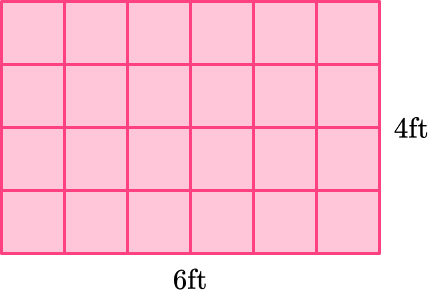The bottom layer of the rectangular prism is 6 by 4 cubes, so it is made up of 24 feet cubes or 24 \, \text {ft}^3.

The height is 4 \, feet , so there are 4 layers of cubes. Since each layer has a volume of 24 \, \text {ft}^3 , you can add the volume of each layer to find the total volume.\begin{aligned} \text {Volume } & =24 \, f t^3+24 \, f t^3+24 \, f t^3+24 \, f t^3 \\\\ & =96 \, f t^3 \end{aligned}

You can also multiply the area of the base ( \text {length } \times \text { width} ) times the \text {height} .

\begin{aligned} \text {Volume } =24 \, \mathrm{ft}^2 \times 4 \, f t \\\\ & =96 \, \mathrm{ft}^3 \end{aligned}

2. Calculate the volume of the rectangular prism.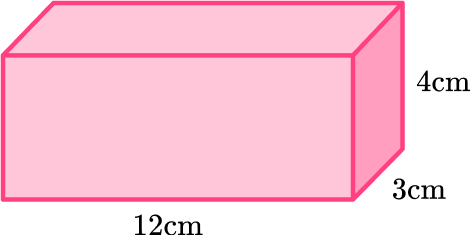144 \, \mathrm{cm}^{2}12 \, \mathrm{cm}^{3}144 \, \mathrm{cm}^{3}36 \, \mathrm{cm}^{3}\begin{aligned} \text{Volume }&= \text{ length }\times \text{ width }\times \text{ height } \\\\ \text{Volume }&= 12 \times 3 \times 4 \\\\ \text{Volume }&= 144 \, \mathrm{cm}^{3} \end{aligned}

3. Calculate the volume of this rectangular prism.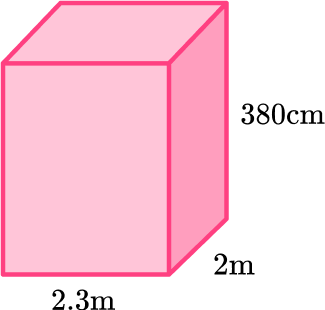1,748 \, \mathrm{cm}^{3}174.8 \, \mathrm{m}^{3}17.48 \, \mathrm{m}^{3}17.48 \, \mathrm{cm}^{3}There are measurements in cm and m , so convert the units before calculating the volume: 380 \, cm to 3.8 \, m .

\begin{aligned} \text{Volume }&= \text{ length }\times \text{ width }\times \text{ height }\\\\ \text{Volume }&= 2.3 \times 2 \times 3.8 \\\\ \text{Volume }&=17.48 \,\mathrm{m}^{3} \end{aligned}

Since the measurements used were in meters, the volume will be in cubic meters.

4. The volume of this rectangular prism is 600 \, cm^{3} . Find the height of the rectangular prism.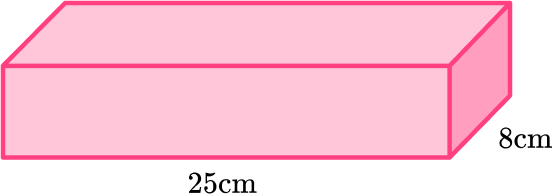3 \, cm24 \, cm200 \, cm75 \, cm\text {Volume }=\text { length } \times \text { width } \times \text { height }

Fill in the known values:

\begin{aligned} & 600=8 \times 25 \times h \\\\ & 600=200 \times h \end{aligned}

The missing height times 200 is equal to 600 , so h=3 \, \mathrm{cm} because 200 \times 3=600 .

5. Calculate the volume of the rectangular prism.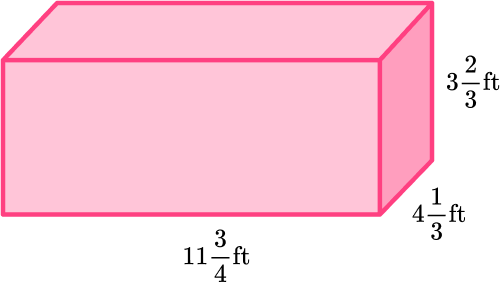186 \, \cfrac{25}{36} \, \mathrm{ft}^3132 \, \cfrac{1}{6} \, \mathrm{ft}^3219 \, \cfrac{7}{9} \, \mathrm{ft}^3360 \, \cfrac{1}{12} \, \mathrm{ft}^3\begin{aligned} & \text {Volume }=\text { length } \times \text { width } \times \text { height } \\\\ & \text {Volume }=11 \, \cfrac{3}{4} \, \times 4 \, \cfrac{1}{3} \, \times 3 \, \cfrac{2}{3} \\\\ & \text {Volume }=\cfrac{47}{4} \, \times \, \cfrac{13}{3} \, \times \, \cfrac{11}{3} \\\\ & \text {Volume }=\cfrac{6,721}{36} \\\\ & \text {Volume }=186 \, \cfrac{25}{36} \, \mathrm{ft}^3 \end{aligned}

6. The base of this prism is a square. The volume of the prism is 450 \, \mathrm{cm}^{3} . Find the height of the prism.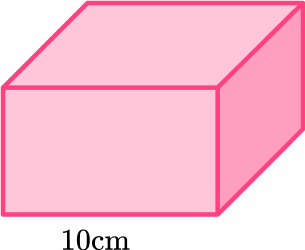45 \, cm4.5 \, cm2.1 \, cm6.7 \, cmSince the base of the prism is a square, the length and the width are both 10 \, cm .

\begin{aligned} & \text {Volume }=\text { length } \times \text { width } \times \text { height } \\\\ & 450=10 \times 10 \times h \\\\ & 450=100 \, h \end{aligned}

The missing height times 100 is equal to 450 , so h=4.5 \, \mathrm{cm} because 100 \times 4.5=450 .

## Volume of a rectangular prism FAQs

What is a cuboid?

A cuboid is a three-dimensional shape with 6 rectangular faces, 8 vertices, and 12 edges. It is another name for a rectangular prism.

What is the volume of a rectangular prism formula?

Volume of a rectangular prism is the area of the base (length times width) times the height of the rectangular prism or l \times w \times h .

Is finding the volume of a triangular prism the same as a rectangular prism?

No, but the formula for both can be stated as the \text {area of the base } \times \text {height of the prism} . However, since there are different formulas for finding the area of a rectangle and finding the area of a triangle, they are not found with the exact same formula.

How do you calculate the surface area of a rectangular prism?

Find the area of the six faces and then add them together.

## Still stuck?

At Third Space Learning, we specialize in helping teachers and school leaders to provide personalized math support for more of their students through high-quality, online one-on-one math tutoring delivered by subject experts.

Each week, our tutors support thousands of students who are at risk of not meeting their grade-level expectations, and help accelerate their progress and boost their confidence.